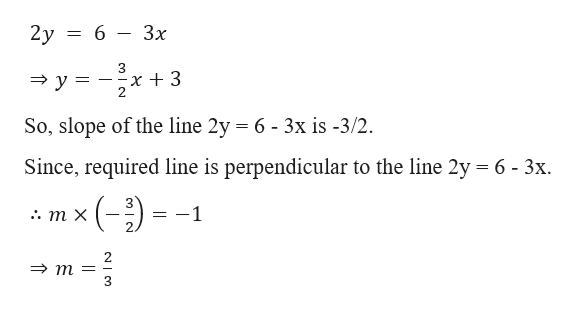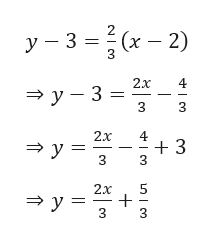# Find the equation of the the line perpendicular to the line (2,3) and 2y= 6-3x

Question
5 views

Find the equation of the the line perpendicular to the line (2,3) and 2y= 6-3x

check_circle

star
star
star
star
star
1 Rating
Step 1

Given,

The required equation of the line passes through the point (2, 3) and perpendicular to the line 2y = 6 - 3x.

Now,

Let the slope of the required line be m.

Now, writing the equation of the line 2y = 6 - 3x in slope-form, we gethelp_outlineImage Transcriptionclose2у %3D 6 Зх x 3 2. 3у 3D So, slope of the line 2y = 6 - 3x is -3/2. Since, required line is perpendicular to the line 2y 6- 3x ()- 1 :. т х m= fullscreen
Step 2

Therefore, required equat...help_outlineImage Transcriptioncloseу -3 %3D (х — 2) 2х 4 —у- 3 %3 3 3 2х 4 3 3у- 3 2х зу с fullscreen

### Want to see the full answer?

See Solution

#### Want to see this answer and more?

Solutions are written by subject experts who are available 24/7. Questions are typically answered within 1 hour.*

See Solution
*Response times may vary by subject and question.
Tagged in

### Equations and In-equations